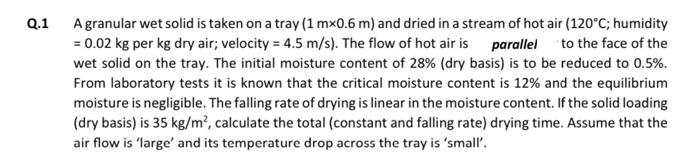# Question Q.1 A granular wet solid is taken on a tray (1 mx0.6 m) and dried in a stream of hot air (120°C; humidity = 0.02 kg per kg dry air; velocity = 4.5 m/s). The flow of hot air is parallel to the face of the wet solid on the tray. The initial moisture content of 28% (dry basis) is to be reduced to 0.5%. From laboratory tests it is known that the critical moisture content is 12% and the equilibrium moisture is negligible. The falling rate of drying is linear in the moisture content. If the solid loading (dry basis) is 35 kg/m², calculate the total (constant and falling rate) drying time. Assume that the air flow is 'large' and its temperature drop across the tray is 'small'.YBVVA4 The Asker · Chemical EngineeringTranscribed Image Text: Q.1 A granular wet solid is taken on a tray (1 mx0.6 m) and dried in a stream of hot air (120°C; humidity = 0.02 kg per kg dry air; velocity = 4.5 m/s). The flow of hot air is parallel to the face of the wet solid on the tray. The initial moisture content of 28% (dry basis) is to be reduced to 0.5%. From laboratory tests it is known that the critical moisture content is 12% and the equilibrium moisture is negligible. The falling rate of drying is linear in the moisture content. If the solid loading (dry basis) is 35 kg/m², calculate the total (constant and falling rate) drying time. Assume that the air flow is 'large' and its temperature drop across the tray is 'small'.
More
Transcribed Image Text: Q.1 A granular wet solid is taken on a tray (1 mx0.6 m) and dried in a stream of hot air (120°C; humidity = 0.02 kg per kg dry air; velocity = 4.5 m/s). The flow of hot air is parallel to the face of the wet solid on the tray. The initial moisture content of 28% (dry basis) is to be reduced to 0.5%. From laboratory tests it is known that the critical moisture content is 12% and the equilibrium moisture is negligible. The falling rate of drying is linear in the moisture content. If the solid loading (dry basis) is 35 kg/m², calculate the total (constant and falling rate) drying time. Assume that the air flow is 'large' and its temperature drop across the tray is 'small'.